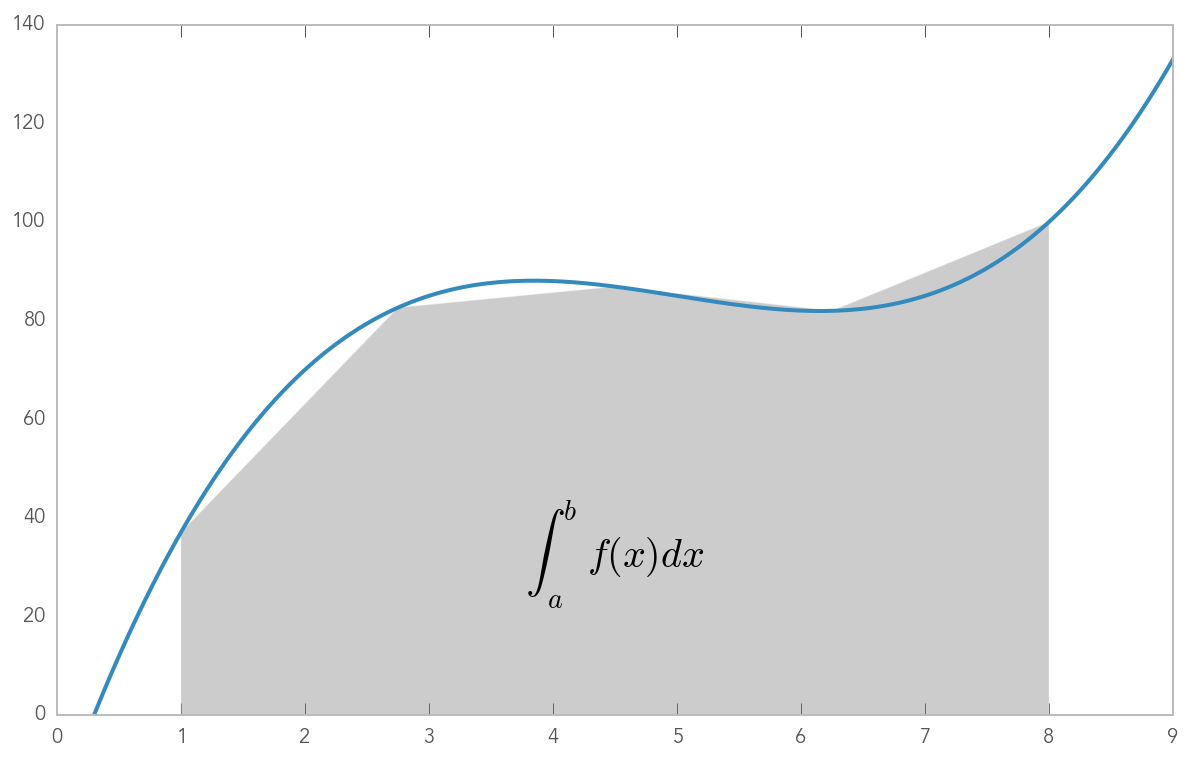Basic Numerical Integration: the Trapezoid Rule¶

A simple illustration of the trapezoid rule for definite integration:

$$\int_{a}^{b} f(x)\, dx \approx \frac{1}{2} \sum_{k=1}^{N} \left( x_{k} - x_{k-1} \right) \left( f(x_{k}) + f(x_{k-1}) \right).$$

First, we define a simple function and sample it between 0 and 10 at 200 points

In :
%matplotlib inline
import numpy as np
import matplotlib.pyplot as plt
In :
def f(x):
return (x-3)*(x-5)*(x-7)+85

x = np.linspace(0, 10, 200)
y = f(x)

Choose a region to integrate over and take only a few points in that region

In :
a, b = 1, 8 # the left and right boundaries
N = 5 # the number of points
xint = np.linspace(a, b, N)
yint = f(xint)

Plot both the function and the area below it in the trapezoid approximation

In :
plt.plot(x, y, lw=2)
plt.axis([0, 9, 0, 140])
plt.fill_between(xint, 0, yint, facecolor='gray', alpha=0.4)
plt.text(0.5 * (a + b), 30,r"$\int_a^b f(x)dx$", horizontalalignment='center', fontsize=20);Compute the integral both at high accuracy and with the trapezoid approximation

In :
from __future__ import print_function
from scipy.integrate import quad
integral, error = quad(f, a, b)
integral_trapezoid = sum( (xint[1:] - xint[:-1]) * (yint[1:] + yint[:-1]) ) / 2
print("The integral is:", integral, "+/-", error)
print("The trapezoid approximation with", len(xint), "points is:", integral_trapezoid)
The integral is: 565.2499999999999 +/- 6.275535646693696e-12
The trapezoid approximation with 5 points is: 559.890625